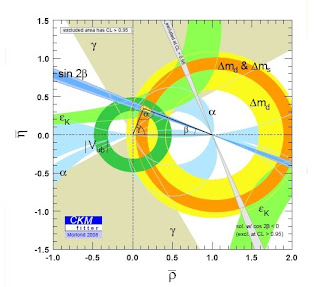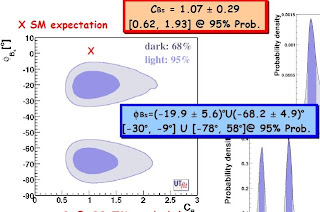## Tuesday, 25 March 2008

### UT fit longo magis quam acri bello

Spring is in the air: birds are singing, trees are blossoming, new physics is popping out from beneath the snow. During this month three different deviations from the Standard Model have been reported, all three in flavour physics:
- 3.8 sigma deviation in leptonic decays of Ds mesons.
- 5.3 sigma deviation of the CP asymmetry in B -> K \pi decays.
- 3 sigma deviation in the phase of B_s mesons mixing.
Sigma's come and go. Yet I suspect the last one on the list may stay longer. Not that I have serious arguments for that - just a hunch slash prejudice. Last Wednesday there was a seminar here at CERN about it: Maurizio Pierini was speaking in the name of the UTfit collaboration
who recently reported the deviation. Maurizio made a very good job explaining what's behind that analysis.The story relates to the transitions between $B_s$ and $\bar B_s$ meson. This is a $\Delta F = 2$ flavour violating process, since a b-quark turns into an anti-b-quark. In the Standard Model, all flavour violation originates from a 3x3 unitary matrix called the CKM matrix. Numerous measurements of the angles and the one physical phase of this matrix resulted in the now-famous LSD plots, considered an important contribution to modern art. A load of results from the B factories led to overconstraining the CKM matrix, and thus to constraining contributions from beyond the Standard Model. New physics in sd transitions (kaon mixing) and bd transitions is tightly constrained. On the other hand, bs transitions are less constrained, basically because B factories were not producing $B_s$ mesons. This gap has been recently filled by the Tevatron who is able to produce $B_s$ mesons and study the $B_s \to J/\Psi \phi$ decay channel. In particular, the mass difference $\Delta m_s$ of the two $B_s$ eigenstates was measured. Furthermore, a constraint on the phase of the mixing $\phi_s$ vs. $\Delta \Gamma$ could be obtained. The latter measurement showed some deviation from the Standard Model prediction, but by itself it was not statistically significant.

New physics at the TeV or higher scale generically contributes to quark transitions between the generations. In the effective theory, where the new physics states are integrated out, we model the effect of the heavy particles by non-renormalizable four-fermion terms in the effective hamiltonian, for example $H_{NP} \sim (\bar b s)^2$. The coefficient of this operator is complex in general. One defines
$C_{B_s} e^{-2 i \phi_{B_s}} = <\bar B_s|H_{SM} + H_{NP}|B_s>/ <\bar B_s|H_{NP}|B_s>$
Analogous parameters are defined for $B_d$ mesons and for kaons. The new parameters affect the same observables as the CKM matrix elements and the new phases are an additional source of CP violation.What the UTfit collaboration did is to add these new parameters to the unitarity triangle fit and to constrain them using all available data, including the Tevatron data on $B_s$ mixing. One complication is that D0 in their analysis employed some assumptions about the strong phases obtained from the $B_d$ sector and UTfit needed to apply some wizardry to disentangle that. The impact of the Tevatron data on the fit is shown on the plot above. The right panel shows the constraints in the $\Delta\Gamma - \phi_s$ plane before taking into account the input from the Tevatron. Both CDF and D0 pick up the region that is away from $\phi_s = 0$. The final result is the constraint on $C_{B_s}$ and $\phi_{B_s}$ shown below. While the amplitude is consistent with the SM value $C_{B_s} = 1$, the phase deviates from 0 at the 3 sigma level.A few points stressed by Maurizio:
- The quoted 3-sigma is not just combining the two 2-sigma deviations reported by CDF and D0, but it's a result of combining their measurements with all other flavour constraints.
- It is highly non-trivial that CDF and D0 results point to the same region of the $\Delta Gamma - \phi_{s}$ plane.
- The determination of the phase is not affected by lattice uncertainties, who only affect $C_{B_s}$.

The situation should clarify in the near future. First of all, D0 should debug their analysis from the strong phases assumptions. The statistics will improve and maybe BELLE will also join the game. If the effect is real it's a fantastic news for LHCb - a Cinderella among the LHC detectors - who will be able to study the $B_s$ sector with much better precision. It'd be funny if flavour were the only place where new physics showed up at the LHC. Such a next-to-nightmare scenario is not so unlikely.

Slides of Maurizio's talk available here. For a thoroughly unenthusiastic discussion of the same results, see another blog.Anonymous said...

maybe mine is a biased prejudice, but anyway: what bit of "standard model prediction" takes into account the systematic uncertainty stemming from bad theory control over hadronic effects? it's hard to accept that the strong phases / hadronic matrix elements involved in such a process as Bs -> J/Psi phi are so well known that one is in a position to actually make a claim....Anonymous said...

Hi jester,

again thank you for the link. I definitely owe you a beer by now.

A comment. The 5.something sigma evidence ppt you point to is all for a fourth generation. Have you seen the latest search results by CDF ? There is a yummy bump at 400 GeV in the t' spectrum... See today's entry in my blog ;-)

Cheers,
T.Anonymous said...

So, who's playing Lucretia here? Systematic uncertainties or theoretical prejudice?

Jester said...

I meant the standard model :-)